# Foundations of Computational Economics #9¶

by Fedor Iskhakov, ANU## Algorithms and complexity¶https://youtu.be/pBbpEBVheOQ

Description: Timing of Python code. Runtime order of growth. Complexity classes. P vs NP.

### Two ways to evaluate the polynomial¶

$$y = a_1 + a_2 x + a_3 x^2 + \dots + a_k x^k$$

Algorithm 1: compute each term, then add together

Algorithm 2: try to avoid computing powers

In :
def calc_polynomial(qs=[0,], x=0.0, algorithm='fast'):
'''Evaluates the polynomial given by coefficients qs at given x.
First coefficient qs is a constant, last coefficient is for highest power.
'''
if algorithm is not 'fast':
# slower algorithm
res=0.0
for k in range(len(qs)):
xpw = x**k
res += qs[k] * xpw
else:
# faster algorithm
res,xpw = qs, x  # init result and power of x
res += xpw * qs[i]
xpw *= x
return res


#### Timing function evaluation¶

Several ways to measure run time in python

• time module
• timeit module (for small snippets)
• profiles (profile and cProfile, for large codes)

In Jupyter Notebooks we can use a magic function timeit

In [ ]:
@timeit <options> <line of code to be timed>

In [ ]:
@@timeit <options> <setup command>
all lines of code in the cell
to be timed together

In :
%%timeit -n100 -r100 qs = [1,]*100
calc_polynomial(qs,15,'slow')

48.3 µs ± 6.72 µs per loop (mean ± std. dev. of 100 runs, 100 loops each)

In :
%%timeit -n100 -r100 qs = [1,]*100
calc_polynomial(qs,15,'fast')

15.6 µs ± 2.33 µs per loop (mean ± std. dev. of 100 runs, 100 loops each)


### Algorithms¶

Sequence of commands for computer to run

1. How much time does it take to run?
2. How much memory does it need?
3. What other resources may be limiting? (storage, communication, etc)

Smart algorithm is a lot more important that fast computer

#### Computational speed and algorithm development¶

Professor Martin Grötschel Konrad-Zuse-Zentrum für Informationstechnik Berlin, expert in optimization

“a benchmark production planning model solved using linear programming would have taken 82 years to solve in 1988, using the computers and the linear programming algorithms of the day. Fifteen years later – in 2003 – this same model could be solved in roughly 1 minute, an improvement by a factor of roughly 43 million. Of this, a factor of roughly 1,000 was due to increased processor speed, whereas a factor of roughly 43,000 was due to improvements in algorithms!”

In :
%%writefile 'algorithm_examples.py'

# Example code to be discussed in the following videos

import time

def parity (n,verbose=False):
'''Returns 1 if passed integer number is odd
'''
if not isinstance(n, int): raise TypeError('Only integers in parity()')
if verbose: print('n = ', format(n, "b"))  # print binary form of the number
return n & 1  # bitwise and operation returns the value of last bit

def maximum_from_list (vars):
'''Returns the maximum from a list of values
'''
m=float('-inf')  # init with the worst value
for v in vars:
if v > m: m = v
return m

def binary_search(grid=[0,1],val=0,delay=0):
'''Returns the index of val on the sorted grid
Optional delay introduces a delay (in microsecond)
'''
i1,i2 = 0,len(grid)-1
if val==grid[i1]: return i1
if val==grid[i2]: return i2
j=(i1+i2)//2
while grid[j]!=val:
if val>grid[j]:
i1=j
else:
i2=j
j=(i1+i2)//2  # divide in half
time.sleep(delay*1e-6)  # micro-sec to seconds
return j

def compositions(N,m):
'''Iterable on compositions of N with m parts
Returns the generator (to be used in for loops)
'''
cmp=[0,]*m
cmp[m-1]=N  # initial composition is all to the last
yield cmp
while cmp!=N:
i=m-1
while cmp[i]==0: i-=1  # find lowest non-zero digit
cmp[i-1] = cmp[i-1]+1  # increment next digit
cmp[m-1] = cmp[i]-1    # the rest to the lowest
if i!=m-1: cmp[i] = 0  # maintain cost sum
yield cmp

Writing algorithm_examples.py


#### Parity of a number¶

Check whether an integer is odd or even.

Algorithm: check the last bit in the binary representation of a number

In :
# import all example code
from algorithm_examples import *

for k in [10**(i+1)+i for i in range(5)]:
print('k=%d (%d bits)' % (k,k.bit_length()))
tt = %timeit -n5000 -r500 -o parity(k)

k=10 (4 bits)
169 ns ± 25.1 ns per loop (mean ± std. dev. of 500 runs, 5000 loops each)
k=101 (7 bits)
171 ns ± 29.8 ns per loop (mean ± std. dev. of 500 runs, 5000 loops each)
k=1002 (10 bits)
170 ns ± 19.8 ns per loop (mean ± std. dev. of 500 runs, 5000 loops each)
k=10003 (14 bits)
173 ns ± 20.3 ns per loop (mean ± std. dev. of 500 runs, 5000 loops each)
k=100004 (17 bits)
172 ns ± 22.3 ns per loop (mean ± std. dev. of 500 runs, 5000 loops each)

In :
import matplotlib.pyplot as plt
%matplotlib inline
plt.rcParams['figure.figsize'] = [12, 8]

N = 50
kk = lambda i: 10**(i+1)+i  # step formula
n,x,std = *N,*N,*N # initialize data lists
for i in range(N):
k = kk(i)  # input value for testing
n[i] = k.bit_length() # size of problem = bits in number
t = %timeit -n5000 -r100 -o parity(k)
x[i] = t.average
std[i] = t.stdev

plt.errorbar(n,x,std)
plt.xlabel('number of bits in the input argument')
plt.ylabel('run time, sec')
plt.show()

172 ns ± 17 ns per loop (mean ± std. dev. of 100 runs, 5000 loops each)
170 ns ± 21.8 ns per loop (mean ± std. dev. of 100 runs, 5000 loops each)
171 ns ± 16.6 ns per loop (mean ± std. dev. of 100 runs, 5000 loops each)
173 ns ± 16.2 ns per loop (mean ± std. dev. of 100 runs, 5000 loops each)
176 ns ± 22.5 ns per loop (mean ± std. dev. of 100 runs, 5000 loops each)
167 ns ± 12.4 ns per loop (mean ± std. dev. of 100 runs, 5000 loops each)
166 ns ± 14.9 ns per loop (mean ± std. dev. of 100 runs, 5000 loops each)
160 ns ± 10.3 ns per loop (mean ± std. dev. of 100 runs, 5000 loops each)
168 ns ± 17.6 ns per loop (mean ± std. dev. of 100 runs, 5000 loops each)
168 ns ± 18.5 ns per loop (mean ± std. dev. of 100 runs, 5000 loops each)
164 ns ± 14.6 ns per loop (mean ± std. dev. of 100 runs, 5000 loops each)
162 ns ± 12.2 ns per loop (mean ± std. dev. of 100 runs, 5000 loops each)
161 ns ± 16.7 ns per loop (mean ± std. dev. of 100 runs, 5000 loops each)
159 ns ± 22.5 ns per loop (mean ± std. dev. of 100 runs, 5000 loops each)
164 ns ± 22.8 ns per loop (mean ± std. dev. of 100 runs, 5000 loops each)
154 ns ± 14.1 ns per loop (mean ± std. dev. of 100 runs, 5000 loops each)
189 ns ± 36.9 ns per loop (mean ± std. dev. of 100 runs, 5000 loops each)
158 ns ± 10.7 ns per loop (mean ± std. dev. of 100 runs, 5000 loops each)
173 ns ± 33.4 ns per loop (mean ± std. dev. of 100 runs, 5000 loops each)
170 ns ± 27.4 ns per loop (mean ± std. dev. of 100 runs, 5000 loops each)
160 ns ± 16.1 ns per loop (mean ± std. dev. of 100 runs, 5000 loops each)
163 ns ± 18.1 ns per loop (mean ± std. dev. of 100 runs, 5000 loops each)
159 ns ± 14.9 ns per loop (mean ± std. dev. of 100 runs, 5000 loops each)
160 ns ± 16.2 ns per loop (mean ± std. dev. of 100 runs, 5000 loops each)
157 ns ± 14.9 ns per loop (mean ± std. dev. of 100 runs, 5000 loops each)
161 ns ± 14.4 ns per loop (mean ± std. dev. of 100 runs, 5000 loops each)
166 ns ± 15.3 ns per loop (mean ± std. dev. of 100 runs, 5000 loops each)
166 ns ± 14.5 ns per loop (mean ± std. dev. of 100 runs, 5000 loops each)
177 ns ± 28 ns per loop (mean ± std. dev. of 100 runs, 5000 loops each)
169 ns ± 15.2 ns per loop (mean ± std. dev. of 100 runs, 5000 loops each)
163 ns ± 13.3 ns per loop (mean ± std. dev. of 100 runs, 5000 loops each)
165 ns ± 17.2 ns per loop (mean ± std. dev. of 100 runs, 5000 loops each)
170 ns ± 16.9 ns per loop (mean ± std. dev. of 100 runs, 5000 loops each)
162 ns ± 12.3 ns per loop (mean ± std. dev. of 100 runs, 5000 loops each)
164 ns ± 16.4 ns per loop (mean ± std. dev. of 100 runs, 5000 loops each)
166 ns ± 13 ns per loop (mean ± std. dev. of 100 runs, 5000 loops each)
166 ns ± 11.2 ns per loop (mean ± std. dev. of 100 runs, 5000 loops each)
163 ns ± 21.3 ns per loop (mean ± std. dev. of 100 runs, 5000 loops each)
173 ns ± 21 ns per loop (mean ± std. dev. of 100 runs, 5000 loops each)
165 ns ± 12.5 ns per loop (mean ± std. dev. of 100 runs, 5000 loops each)
184 ns ± 27.2 ns per loop (mean ± std. dev. of 100 runs, 5000 loops each)
175 ns ± 22.7 ns per loop (mean ± std. dev. of 100 runs, 5000 loops each)
163 ns ± 11.1 ns per loop (mean ± std. dev. of 100 runs, 5000 loops each)
161 ns ± 13.7 ns per loop (mean ± std. dev. of 100 runs, 5000 loops each)
171 ns ± 17.9 ns per loop (mean ± std. dev. of 100 runs, 5000 loops each)
168 ns ± 15 ns per loop (mean ± std. dev. of 100 runs, 5000 loops each)
161 ns ± 13 ns per loop (mean ± std. dev. of 100 runs, 5000 loops each)
164 ns ± 19.8 ns per loop (mean ± std. dev. of 100 runs, 5000 loops each)
164 ns ± 13.7 ns per loop (mean ± std. dev. of 100 runs, 5000 loops each)
173 ns ± 37.9 ns per loop (mean ± std. dev. of 100 runs, 5000 loops each)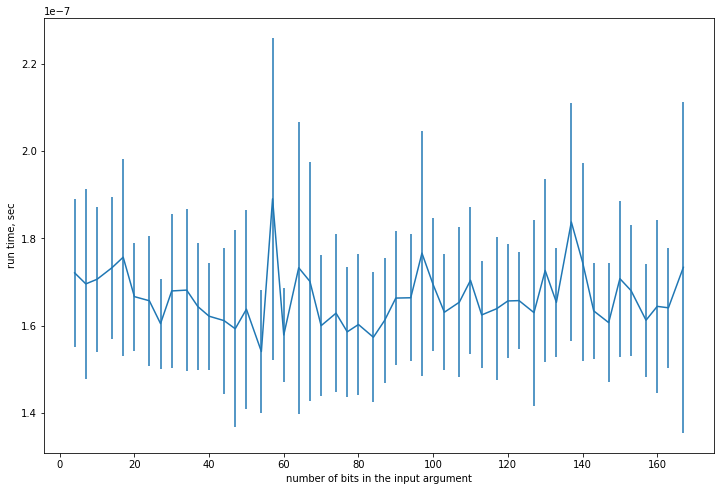#### Finding max/min of a list¶

Find max or min in an unsorted list of values

Algorithm: run through the list once

In :
import numpy as np

N = 10
# generate uniformly distributed values between given bounds
x = np.random.uniform(low=0.0, high=100.0, size=N)
print(x)
print("max=%f"%maximum_from_list(x))

[ 9.79874372  0.2020611   1.00212784 43.00195447 13.94713462 12.22406642
6.3382257  68.06549963 56.02208578 10.6578756 ]
max=68.065500

In :
N = 50
kk = lambda i: 2*i  # step formula
n,x,std = *N,*N,*N # initialize data lists
for i in range(N):
n[i] = kk(i) # size of the array
vv = np.random.uniform(low=0.0, high=100.0, size=n[i])
t = %timeit -n1000 -r100 -o maximum_from_list(vv)
x[i] = t.average
std[i] = t.stdev

plt.errorbar(n,x,std)
plt.xlabel('number of elements in the list')
plt.ylabel('run time, sec')
plt.show()

584 ns ± 71.1 ns per loop (mean ± std. dev. of 100 runs, 1000 loops each)
826 ns ± 99 ns per loop (mean ± std. dev. of 100 runs, 1000 loops each)
1.08 µs ± 209 ns per loop (mean ± std. dev. of 100 runs, 1000 loops each)
1.2 µs ± 152 ns per loop (mean ± std. dev. of 100 runs, 1000 loops each)
1.4 µs ± 143 ns per loop (mean ± std. dev. of 100 runs, 1000 loops each)
1.66 µs ± 231 ns per loop (mean ± std. dev. of 100 runs, 1000 loops each)
1.85 µs ± 178 ns per loop (mean ± std. dev. of 100 runs, 1000 loops each)
2.3 µs ± 286 ns per loop (mean ± std. dev. of 100 runs, 1000 loops each)
2.2 µs ± 160 ns per loop (mean ± std. dev. of 100 runs, 1000 loops each)
2.44 µs ± 175 ns per loop (mean ± std. dev. of 100 runs, 1000 loops each)
2.47 µs ± 270 ns per loop (mean ± std. dev. of 100 runs, 1000 loops each)
2.83 µs ± 292 ns per loop (mean ± std. dev. of 100 runs, 1000 loops each)
2.86 µs ± 182 ns per loop (mean ± std. dev. of 100 runs, 1000 loops each)
3.29 µs ± 400 ns per loop (mean ± std. dev. of 100 runs, 1000 loops each)
3.49 µs ± 419 ns per loop (mean ± std. dev. of 100 runs, 1000 loops each)
3.69 µs ± 305 ns per loop (mean ± std. dev. of 100 runs, 1000 loops each)
3.69 µs ± 355 ns per loop (mean ± std. dev. of 100 runs, 1000 loops each)
3.84 µs ± 311 ns per loop (mean ± std. dev. of 100 runs, 1000 loops each)
4.32 µs ± 588 ns per loop (mean ± std. dev. of 100 runs, 1000 loops each)
4.35 µs ± 445 ns per loop (mean ± std. dev. of 100 runs, 1000 loops each)
4.29 µs ± 411 ns per loop (mean ± std. dev. of 100 runs, 1000 loops each)
4.43 µs ± 476 ns per loop (mean ± std. dev. of 100 runs, 1000 loops each)
4.51 µs ± 324 ns per loop (mean ± std. dev. of 100 runs, 1000 loops each)
4.7 µs ± 137 ns per loop (mean ± std. dev. of 100 runs, 1000 loops each)
6 µs ± 547 ns per loop (mean ± std. dev. of 100 runs, 1000 loops each)
5.43 µs ± 638 ns per loop (mean ± std. dev. of 100 runs, 1000 loops each)
5.35 µs ± 412 ns per loop (mean ± std. dev. of 100 runs, 1000 loops each)
6.69 µs ± 421 ns per loop (mean ± std. dev. of 100 runs, 1000 loops each)
6.13 µs ± 643 ns per loop (mean ± std. dev. of 100 runs, 1000 loops each)
5.96 µs ± 557 ns per loop (mean ± std. dev. of 100 runs, 1000 loops each)
6.06 µs ± 527 ns per loop (mean ± std. dev. of 100 runs, 1000 loops each)
6.26 µs ± 271 ns per loop (mean ± std. dev. of 100 runs, 1000 loops each)
6.14 µs ± 365 ns per loop (mean ± std. dev. of 100 runs, 1000 loops each)
6.59 µs ± 403 ns per loop (mean ± std. dev. of 100 runs, 1000 loops each)
7.17 µs ± 536 ns per loop (mean ± std. dev. of 100 runs, 1000 loops each)
6.66 µs ± 348 ns per loop (mean ± std. dev. of 100 runs, 1000 loops each)
6.85 µs ± 465 ns per loop (mean ± std. dev. of 100 runs, 1000 loops each)
7.21 µs ± 422 ns per loop (mean ± std. dev. of 100 runs, 1000 loops each)
7.26 µs ± 394 ns per loop (mean ± std. dev. of 100 runs, 1000 loops each)
7.71 µs ± 757 ns per loop (mean ± std. dev. of 100 runs, 1000 loops each)
7.61 µs ± 550 ns per loop (mean ± std. dev. of 100 runs, 1000 loops each)
7.8 µs ± 484 ns per loop (mean ± std. dev. of 100 runs, 1000 loops each)
7.86 µs ± 606 ns per loop (mean ± std. dev. of 100 runs, 1000 loops each)
8.07 µs ± 548 ns per loop (mean ± std. dev. of 100 runs, 1000 loops each)
8.2 µs ± 406 ns per loop (mean ± std. dev. of 100 runs, 1000 loops each)
9.07 µs ± 1.01 µs per loop (mean ± std. dev. of 100 runs, 1000 loops each)
10.2 µs ± 1.19 µs per loop (mean ± std. dev. of 100 runs, 1000 loops each)
10.1 µs ± 933 ns per loop (mean ± std. dev. of 100 runs, 1000 loops each)
10.1 µs ± 967 ns per loop (mean ± std. dev. of 100 runs, 1000 loops each)
9.63 µs ± 544 ns per loop (mean ± std. dev. of 100 runs, 1000 loops each)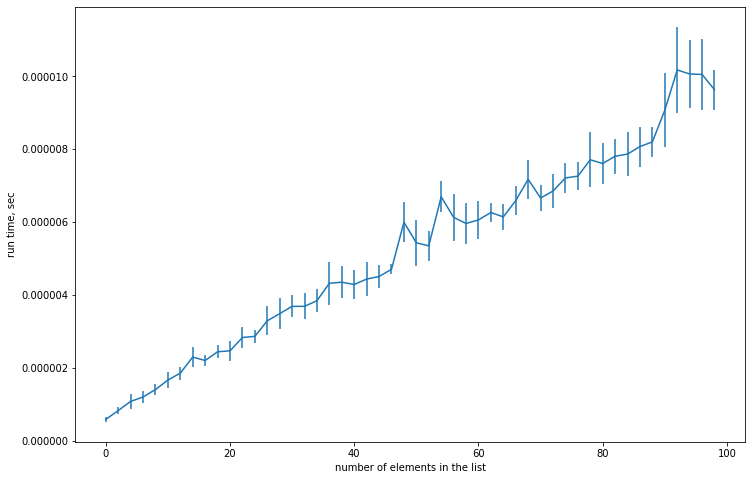Find an element between given boundaries

1. Think of number between 1 and 100
2. How many guesses are needed to locate it if the only answers are “below” and “above”?
3. What is the optimal sequece of questions?
In :
N = 10
# random sorted sequence of integers up to 100
x = np.random.choice(100,size=N,replace=False)
x = np.sort(x)
# random choice of one number/index
k0 = np.random.choice(N,size=1)

k1 = binary_search(grid=x,val=x[k0])
print("Index of x[%d]=%d in %r is %d"%(k0,x[k0],x,k1))

Index of x=78 in array([ 8, 14, 24, 29, 34, 42, 43, 54, 78, 99]) is 8

In :
N = 50  # number of points
kk = lambda i: 100+(i+1)*500  # step formula
# precompute the sorted sequence of integers of max length
vv = np.random.choice(10*kk(N),size=kk(N),replace=False)
vv = np.sort(vv)

n,x,std = *N,*N,*N   # initialize lists
for i in range(N):
n[i] = kk(i)  # number of list elements
# randomize the choice in each run to smooth out simulation error
t = %timeit -n10 -r100 -o binary_search(grid=vv[:n[i]],val=vv[np.random.choice(n[i],size=1)],delay=50)
x[i] = t.average
std[i] = t.stdev

plt.errorbar(n,x,std)
plt.xlabel('number of elements in the list')
plt.ylabel('run time, sec')
plt.show()

plt.errorbar(n,x,std)
plt.xscale('log')
plt.xlabel('log(number of elements in the list)')
plt.ylabel('run time, sec')
plt.show()

577 µs ± 60.9 µs per loop (mean ± std. dev. of 100 runs, 10 loops each)
637 µs ± 48.2 µs per loop (mean ± std. dev. of 100 runs, 10 loops each)
733 µs ± 77.9 µs per loop (mean ± std. dev. of 100 runs, 10 loops each)
775 µs ± 108 µs per loop (mean ± std. dev. of 100 runs, 10 loops each)
794 µs ± 72.3 µs per loop (mean ± std. dev. of 100 runs, 10 loops each)
865 µs ± 81.2 µs per loop (mean ± std. dev. of 100 runs, 10 loops each)
897 µs ± 77.5 µs per loop (mean ± std. dev. of 100 runs, 10 loops each)
829 µs ± 88.7 µs per loop (mean ± std. dev. of 100 runs, 10 loops each)
840 µs ± 105 µs per loop (mean ± std. dev. of 100 runs, 10 loops each)
846 µs ± 65.3 µs per loop (mean ± std. dev. of 100 runs, 10 loops each)
830 µs ± 70.3 µs per loop (mean ± std. dev. of 100 runs, 10 loops each)
852 µs ± 76.4 µs per loop (mean ± std. dev. of 100 runs, 10 loops each)
864 µs ± 91.4 µs per loop (mean ± std. dev. of 100 runs, 10 loops each)
883 µs ± 108 µs per loop (mean ± std. dev. of 100 runs, 10 loops each)
870 µs ± 85.9 µs per loop (mean ± std. dev. of 100 runs, 10 loops each)
859 µs ± 69.5 µs per loop (mean ± std. dev. of 100 runs, 10 loops each)
886 µs ± 96.4 µs per loop (mean ± std. dev. of 100 runs, 10 loops each)
987 µs ± 106 µs per loop (mean ± std. dev. of 100 runs, 10 loops each)
903 µs ± 86 µs per loop (mean ± std. dev. of 100 runs, 10 loops each)
868 µs ± 41.1 µs per loop (mean ± std. dev. of 100 runs, 10 loops each)
884 µs ± 44.8 µs per loop (mean ± std. dev. of 100 runs, 10 loops each)
944 µs ± 100 µs per loop (mean ± std. dev. of 100 runs, 10 loops each)
927 µs ± 111 µs per loop (mean ± std. dev. of 100 runs, 10 loops each)
898 µs ± 59.3 µs per loop (mean ± std. dev. of 100 runs, 10 loops each)
896 µs ± 43.4 µs per loop (mean ± std. dev. of 100 runs, 10 loops each)
897 µs ± 45.6 µs per loop (mean ± std. dev. of 100 runs, 10 loops each)
896 µs ± 48.9 µs per loop (mean ± std. dev. of 100 runs, 10 loops each)
915 µs ± 52.2 µs per loop (mean ± std. dev. of 100 runs, 10 loops each)
1 ms ± 126 µs per loop (mean ± std. dev. of 100 runs, 10 loops each)
934 µs ± 65 µs per loop (mean ± std. dev. of 100 runs, 10 loops each)
940 µs ± 74.4 µs per loop (mean ± std. dev. of 100 runs, 10 loops each)
936 µs ± 82.8 µs per loop (mean ± std. dev. of 100 runs, 10 loops each)
944 µs ± 65.6 µs per loop (mean ± std. dev. of 100 runs, 10 loops each)
959 µs ± 91.1 µs per loop (mean ± std. dev. of 100 runs, 10 loops each)
974 µs ± 95.5 µs per loop (mean ± std. dev. of 100 runs, 10 loops each)
981 µs ± 111 µs per loop (mean ± std. dev. of 100 runs, 10 loops each)
1.06 ms ± 89.9 µs per loop (mean ± std. dev. of 100 runs, 10 loops each)
960 µs ± 77.6 µs per loop (mean ± std. dev. of 100 runs, 10 loops each)
976 µs ± 94.3 µs per loop (mean ± std. dev. of 100 runs, 10 loops each)
976 µs ± 108 µs per loop (mean ± std. dev. of 100 runs, 10 loops each)
981 µs ± 94.9 µs per loop (mean ± std. dev. of 100 runs, 10 loops each)
1.01 ms ± 90.2 µs per loop (mean ± std. dev. of 100 runs, 10 loops each)
1.04 ms ± 106 µs per loop (mean ± std. dev. of 100 runs, 10 loops each)
973 µs ± 98.3 µs per loop (mean ± std. dev. of 100 runs, 10 loops each)
1.09 ms ± 113 µs per loop (mean ± std. dev. of 100 runs, 10 loops each)
1.07 ms ± 112 µs per loop (mean ± std. dev. of 100 runs, 10 loops each)
1.05 ms ± 122 µs per loop (mean ± std. dev. of 100 runs, 10 loops each)
991 µs ± 74.9 µs per loop (mean ± std. dev. of 100 runs, 10 loops each)
1 ms ± 72.6 µs per loop (mean ± std. dev. of 100 runs, 10 loops each)
993 µs ± 89.6 µs per loop (mean ± std. dev. of 100 runs, 10 loops each)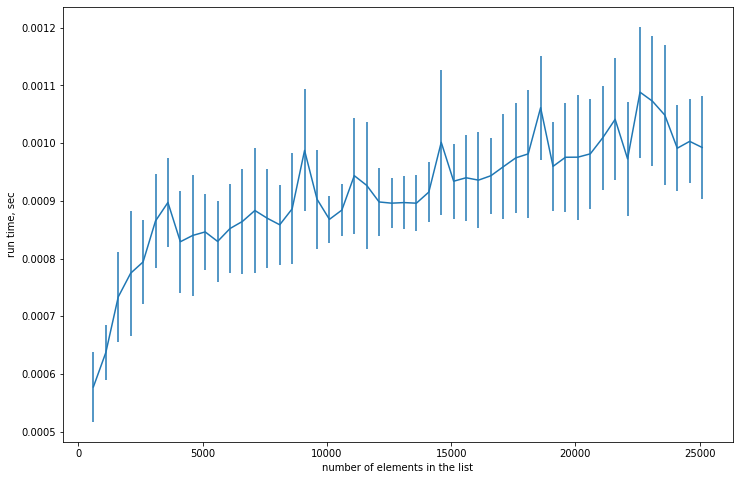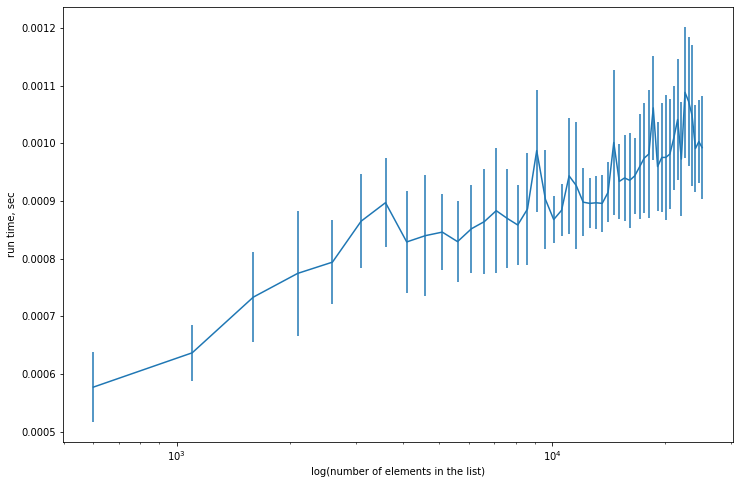#### Binary tree and divide-and-conquer algorithms¶### Big-O notation¶

Useful way to label the complexity of an algorithm, where $n$ is the size of the input or other dimension of the problem

$$f(n)=\mathcal{O}\big(g(n)\big) \Leftrightarrow$$$$\exists M>0 \text{ and } N \text{ such than } |f(n)| < M |g(n)| \text{ for all } n>N,$$

Thus, functions $f(n)$ and $g(n)$ grow on the same order

### Focus on the worst case¶

In measuring solution time we may distinguish performance in

• best (easiest to solve) case
• average case
• worst case ($\leftarrow$ the focus of the theory!)

Constants and lower terms are ignored because we are only interested in order or growth

### Classes of algorithm complexity¶

• $\mathcal{O}(1)$ constant time
• $\mathcal{O}(\log_{2}(n))$ logarithmic time
• $\mathcal{O}(n)$ linear time
• $\mathcal{O}(n \log_{2}(n))$ quasi-linear time
• $\mathcal{O}(n^{k}), k>1$ quadratic, cubic, etc. polinomial time $\uparrow$ Tractable
• $\mathcal{O}(2^{n})$ exponential time $\downarrow$ Curse of dimensionality
• $\mathcal{O}(n!)$ factorial time

#### Classes of algorithm complexity¶#### How many operations as function of input size?¶

• Parity: Just need to check the lowest bit, does not depend on input size $\Rightarrow \mathcal{O}(1)$
• Maximum element: Need to loop through elements once: $\Rightarrow \mathcal{O}(n)$
• Binary search: Divide the problem in 2 each step $\Rightarrow \mathcal{O}(\log(n))$
• Examples of $\mathcal{O}(2^n)$ or more?

#### Allocation of discrete good¶

Problem

Maximize welfare $W(x_1,x_2,\dots,x_n)$ subject to $\sum_{i=1}^{n}x_i = A$ where $A$ is discrete good that is only divisible in steps of $\Lambda$.

Let $M=A/\Lambda \in \mathbb{N}$. Let $p_i \in \{0,1,\dots,M\}$ such that $\sum_{i=1}^{n}p_i = M$.

Then the problem is equivalent to maximize $W(\Lambda p_1,\Lambda p_2,\dots,\Lambda p_n)$ subject to above.

$(p_1,p_2,\dots,p_n)$ is composition of number $M$ into $n$ parts.

In :
import scipy.special
n, M = 4, 8
total = scipy.special.comb(M+n-1,n-1) # M+n-1 choose n-1
print("Total number of compositions is %d"%total)

for i,k in enumerate(compositions(M,n)):
print('%3d'%i,end=": ")
print(k)

Total number of compositions is 165
0: [0, 0, 0, 8]
1: [0, 0, 1, 7]
2: [0, 0, 2, 6]
3: [0, 0, 3, 5]
4: [0, 0, 4, 4]
5: [0, 0, 5, 3]
6: [0, 0, 6, 2]
7: [0, 0, 7, 1]
8: [0, 0, 8, 0]
9: [0, 1, 0, 7]
10: [0, 1, 1, 6]
11: [0, 1, 2, 5]
12: [0, 1, 3, 4]
13: [0, 1, 4, 3]
14: [0, 1, 5, 2]
15: [0, 1, 6, 1]
16: [0, 1, 7, 0]
17: [0, 2, 0, 6]
18: [0, 2, 1, 5]
19: [0, 2, 2, 4]
20: [0, 2, 3, 3]
21: [0, 2, 4, 2]
22: [0, 2, 5, 1]
23: [0, 2, 6, 0]
24: [0, 3, 0, 5]
25: [0, 3, 1, 4]
26: [0, 3, 2, 3]
27: [0, 3, 3, 2]
28: [0, 3, 4, 1]
29: [0, 3, 5, 0]
30: [0, 4, 0, 4]
31: [0, 4, 1, 3]
32: [0, 4, 2, 2]
33: [0, 4, 3, 1]
34: [0, 4, 4, 0]
35: [0, 5, 0, 3]
36: [0, 5, 1, 2]
37: [0, 5, 2, 1]
38: [0, 5, 3, 0]
39: [0, 6, 0, 2]
40: [0, 6, 1, 1]
41: [0, 6, 2, 0]
42: [0, 7, 0, 1]
43: [0, 7, 1, 0]
44: [0, 8, 0, 0]
45: [1, 0, 0, 7]
46: [1, 0, 1, 6]
47: [1, 0, 2, 5]
48: [1, 0, 3, 4]
49: [1, 0, 4, 3]
50: [1, 0, 5, 2]
51: [1, 0, 6, 1]
52: [1, 0, 7, 0]
53: [1, 1, 0, 6]
54: [1, 1, 1, 5]
55: [1, 1, 2, 4]
56: [1, 1, 3, 3]
57: [1, 1, 4, 2]
58: [1, 1, 5, 1]
59: [1, 1, 6, 0]
60: [1, 2, 0, 5]
61: [1, 2, 1, 4]
62: [1, 2, 2, 3]
63: [1, 2, 3, 2]
64: [1, 2, 4, 1]
65: [1, 2, 5, 0]
66: [1, 3, 0, 4]
67: [1, 3, 1, 3]
68: [1, 3, 2, 2]
69: [1, 3, 3, 1]
70: [1, 3, 4, 0]
71: [1, 4, 0, 3]
72: [1, 4, 1, 2]
73: [1, 4, 2, 1]
74: [1, 4, 3, 0]
75: [1, 5, 0, 2]
76: [1, 5, 1, 1]
77: [1, 5, 2, 0]
78: [1, 6, 0, 1]
79: [1, 6, 1, 0]
80: [1, 7, 0, 0]
81: [2, 0, 0, 6]
82: [2, 0, 1, 5]
83: [2, 0, 2, 4]
84: [2, 0, 3, 3]
85: [2, 0, 4, 2]
86: [2, 0, 5, 1]
87: [2, 0, 6, 0]
88: [2, 1, 0, 5]
89: [2, 1, 1, 4]
90: [2, 1, 2, 3]
91: [2, 1, 3, 2]
92: [2, 1, 4, 1]
93: [2, 1, 5, 0]
94: [2, 2, 0, 4]
95: [2, 2, 1, 3]
96: [2, 2, 2, 2]
97: [2, 2, 3, 1]
98: [2, 2, 4, 0]
99: [2, 3, 0, 3]
100: [2, 3, 1, 2]
101: [2, 3, 2, 1]
102: [2, 3, 3, 0]
103: [2, 4, 0, 2]
104: [2, 4, 1, 1]
105: [2, 4, 2, 0]
106: [2, 5, 0, 1]
107: [2, 5, 1, 0]
108: [2, 6, 0, 0]
109: [3, 0, 0, 5]
110: [3, 0, 1, 4]
111: [3, 0, 2, 3]
112: [3, 0, 3, 2]
113: [3, 0, 4, 1]
114: [3, 0, 5, 0]
115: [3, 1, 0, 4]
116: [3, 1, 1, 3]
117: [3, 1, 2, 2]
118: [3, 1, 3, 1]
119: [3, 1, 4, 0]
120: [3, 2, 0, 3]
121: [3, 2, 1, 2]
122: [3, 2, 2, 1]
123: [3, 2, 3, 0]
124: [3, 3, 0, 2]
125: [3, 3, 1, 1]
126: [3, 3, 2, 0]
127: [3, 4, 0, 1]
128: [3, 4, 1, 0]
129: [3, 5, 0, 0]
130: [4, 0, 0, 4]
131: [4, 0, 1, 3]
132: [4, 0, 2, 2]
133: [4, 0, 3, 1]
134: [4, 0, 4, 0]
135: [4, 1, 0, 3]
136: [4, 1, 1, 2]
137: [4, 1, 2, 1]
138: [4, 1, 3, 0]
139: [4, 2, 0, 2]
140: [4, 2, 1, 1]
141: [4, 2, 2, 0]
142: [4, 3, 0, 1]
143: [4, 3, 1, 0]
144: [4, 4, 0, 0]
145: [5, 0, 0, 3]
146: [5, 0, 1, 2]
147: [5, 0, 2, 1]
148: [5, 0, 3, 0]
149: [5, 1, 0, 2]
150: [5, 1, 1, 1]
151: [5, 1, 2, 0]
152: [5, 2, 0, 1]
153: [5, 2, 1, 0]
154: [5, 3, 0, 0]
155: [6, 0, 0, 2]
156: [6, 0, 1, 1]
157: [6, 0, 2, 0]
158: [6, 1, 0, 1]
159: [6, 1, 1, 0]
160: [6, 2, 0, 0]
161: [7, 0, 0, 1]
162: [7, 0, 1, 0]
163: [7, 1, 0, 0]
164: [8, 0, 0, 0]

In :
N = 10  # number of points
kk = lambda i: 2+i  # step formula
M = 20  # quantity of indivisible good in units of lambda

n,x,std = *N,*N,*N   # initialize lists
for i in range(N):
n[i] = kk(i)  # number of list elements
t = %timeit -n2 -r10 -o for c in compositions(M,n[i]): pass
x[i] = t.average
std[i] = t.stdev

plt.errorbar(n,x,std)
plt.xlabel('Number of elements in compositions')
plt.ylabel('run time, sec')
plt.show()

plt.errorbar(n,x,std)
plt.yscale('log')
plt.xlabel('Number of elements in compositions')
plt.ylabel('log(run time)')
plt.show()

5.69 µs ± 298 ns per loop (mean ± std. dev. of 10 runs, 2 loops each)
60.1 µs ± 398 ns per loop (mean ± std. dev. of 10 runs, 2 loops each)
515 µs ± 120 µs per loop (mean ± std. dev. of 10 runs, 2 loops each)
3.02 ms ± 184 µs per loop (mean ± std. dev. of 10 runs, 2 loops each)
16.7 ms ± 1.01 ms per loop (mean ± std. dev. of 10 runs, 2 loops each)
71.1 ms ± 3.83 ms per loop (mean ± std. dev. of 10 runs, 2 loops each)
250 ms ± 3.04 ms per loop (mean ± std. dev. of 10 runs, 2 loops each)
893 ms ± 18.4 ms per loop (mean ± std. dev. of 10 runs, 2 loops each)
2.96 s ± 90.7 ms per loop (mean ± std. dev. of 10 runs, 2 loops each)
8.83 s ± 122 ms per loop (mean ± std. dev. of 10 runs, 2 loops each)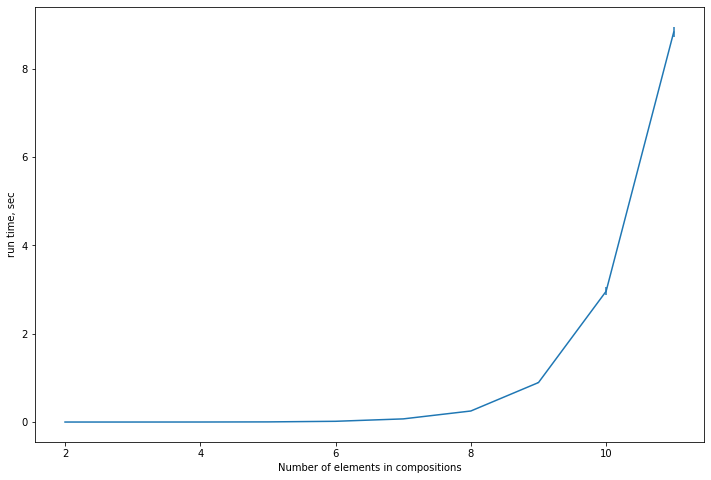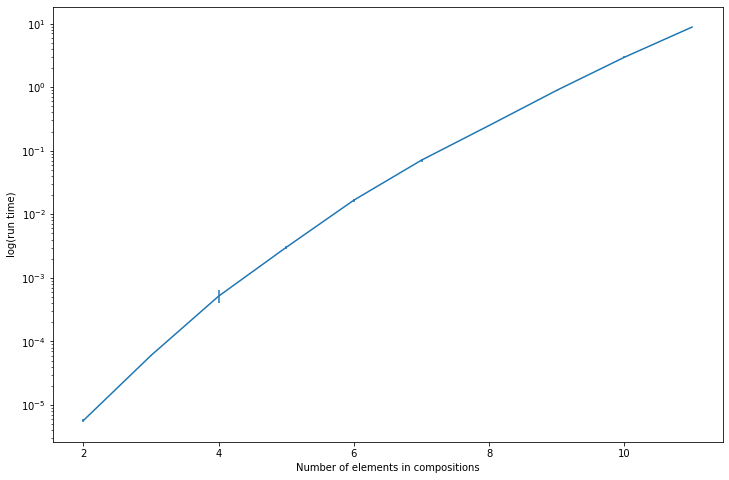#### Other exponential algorithms¶

• Many board games (checkers, chess, shogi, go) in n-by-n generalizations
• Traveling salesman problem (TSP)
• Many problems in economics are subject to “curse of dimensionality”

Curse of dimensionality = exponential time in solution algorithms

#### What to do with heavy to compute models?¶

1. Design of better solution algorithms
2. Analyze special classes of problems + rely on problem structure
3. Speed up the code (low level language, compilation to machine code)
4. Parallelize the computations
5. Bound the problem to maximize model usefulness while keeping it tractable
6. Wait for innovations in computing technology

#### Classes of computational complexity¶

Thinking of all problems there are:

• P can be solved in polynomial time
• NP solution can checked in polynomial time, even if requires exponential solution algorithm
• NP-hard as complex as any NP problem (including all exponential and combinatorial problems)
• NP-complete both NP and NP-hard (tied via reductions)

NP stands for non-deterministic polynomial time $\leftrightarrow$ ‘magic’ guess algorithm

#### P vs. NP¶

Unresolved question of whether P = NP or P $\ne$ NP (\\$1 mln. prize by Clay Mathematics Institute)